# Solving Quadratic Equations Square Root Method The square

• Slides: 9Solving Quadratic Equations – Square Root Method • The square root method can be used to solve a quadratic equation that can be set up into the following form: • Examples: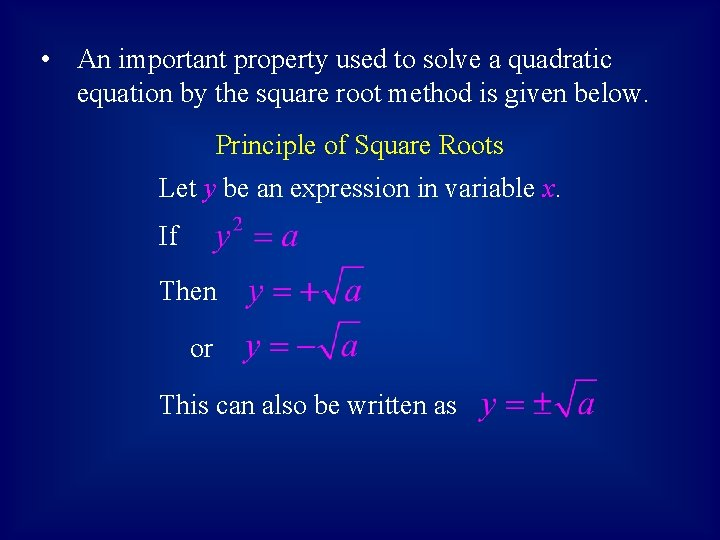• An important property used to solve a quadratic equation by the square root method is given below. Principle of Square Roots Let y be an expression in variable x. If Then or This can also be written as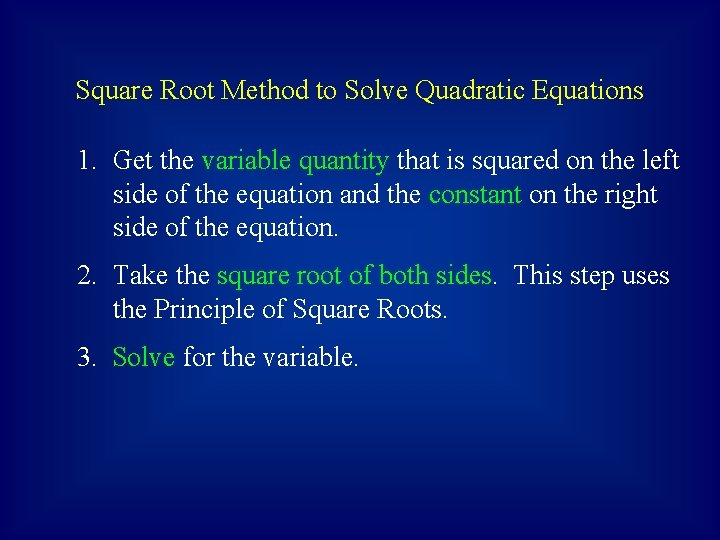Square Root Method to Solve Quadratic Equations 1. Get the variable quantity that is squared on the left side of the equation and the constant on the right side of the equation. 2. Take the square root of both sides. This step uses the Principle of Square Roots. 3. Solve for the variable.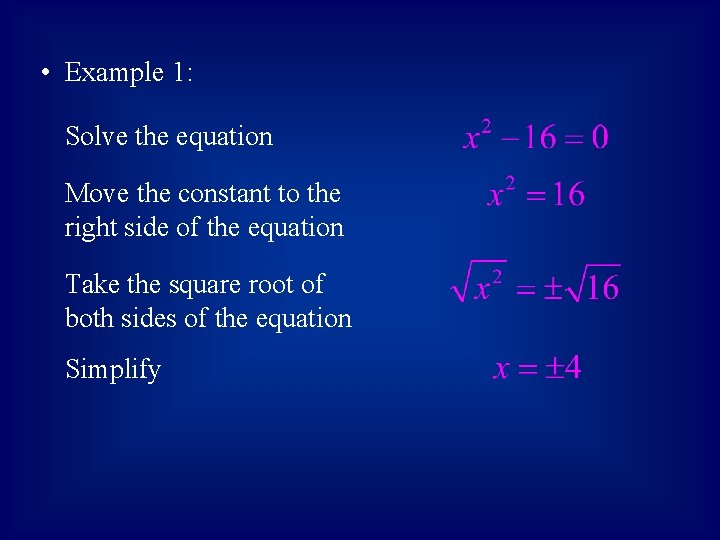• Example 1: Solve the equation Move the constant to the right side of the equation Take the square root of both sides of the equation Simplify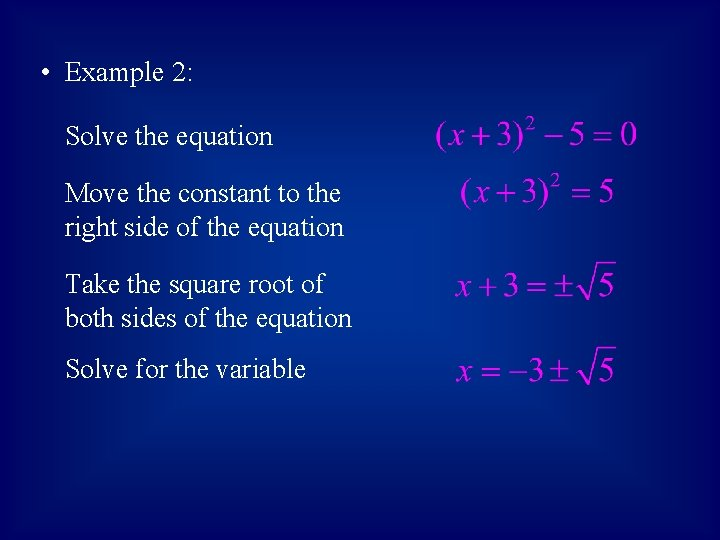• Example 2: Solve the equation Move the constant to the right side of the equation Take the square root of both sides of the equation Solve for the variable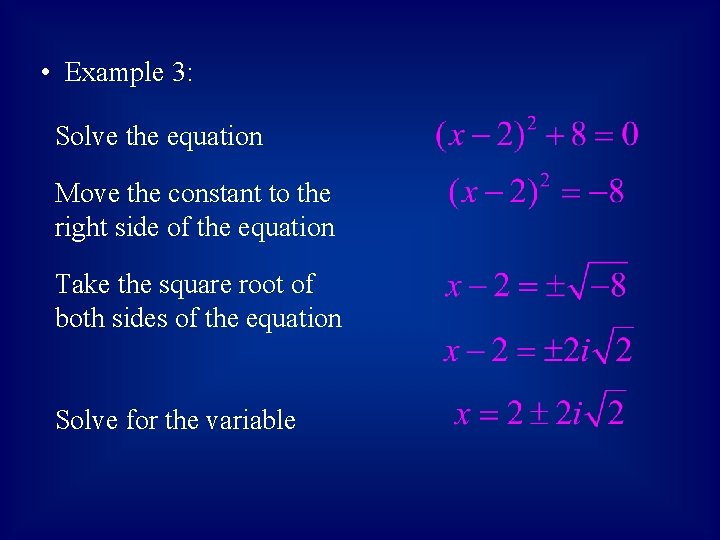• Example 3: Solve the equation Move the constant to the right side of the equation Take the square root of both sides of the equation Solve for the variable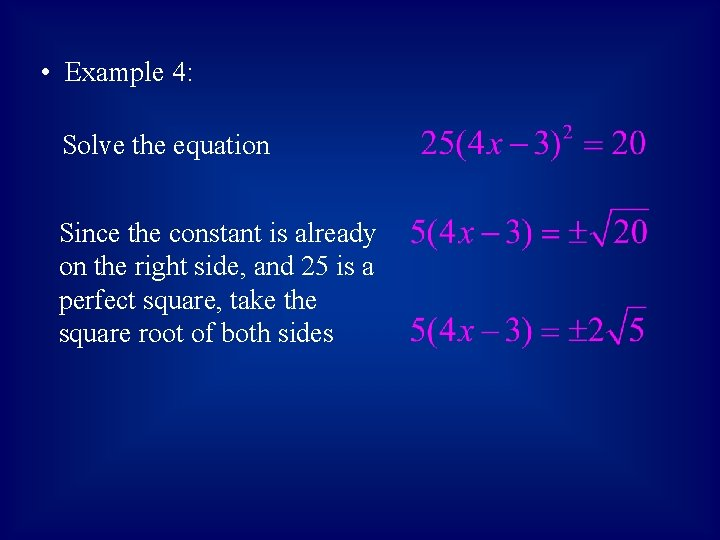• Example 4: Solve the equation Since the constant is already on the right side, and 25 is a perfect square, take the square root of both sidesSolve for the variable